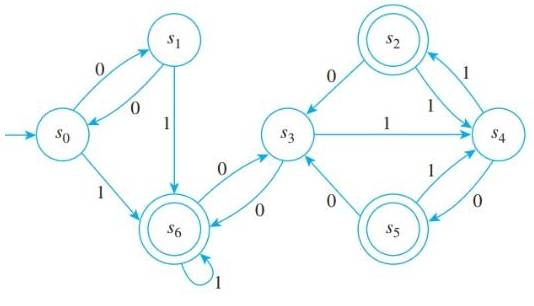Chapter 12.3, Problem 2ES### Discrete Mathematics With Applicat...

5th Edition
EPP + 1 other
ISBN: 9781337694193

#### Solutions

Chapter
Section### Discrete Mathematics With Applicat...

5th Edition
EPP + 1 other
ISBN: 9781337694193
Textbook Problem
11 views

# Consider the finite-state automaton A given by the following transition diagram:a. Find the 0-, 1-, and 2-equivalence classes of states of A. b. Draw the transition diagram for A ¯ , the quotient automaton of A.

To determine

(a)

To find:

The 0-, 1- and 2- equivalence classes of states A.

Explanation

Given information:

The finite-state automaton A given by the following transition diagram:

Definition used:

S is k-equivalent to t if and only if for all input strings w of length less than or equal to k, N*(s,w) and N*(t,w) are both either accepting states or both non-accepting states.

Calculation:

Two states s and t are 0-equivalent If and only if they are both either accepting states or are both non-accepting states.

The 0-equivalent classes are the set of all accepting states and the set of all non-accepting states.

Set of all accepting states ={s2,s5,s6}

Set of all non-accepting states ={s0,s1,s3,s4}

0-equivalent classes ={s2,s5,s6},{s0,s1,s3,s4}

Two states s and t are 1-equivalent If and only if for all input strings w of length less than or equal to 1, N*(s,w) and N*(t,w) are both either accepting states or both non-accepting states.

All strings of length 1 are 0 and 1.

s0 and s1, s2 and s5 are equivalent.

From the given diagram, the 1-equivalentis given by

1-equivalent ={s0,s1},{s2,s5},{s3},{s4},{s6}

Two states s and t are 2-equivalent If and only if for all input strings w of length less than or equal to 2, N*(s,w) and N*(t,w) are both either accepting states or both non-accepting states

To determine

(b)

To draw:

A transition diagram for A¯, the quotient automaton of A.

### Still sussing out bartleby?

Check out a sample textbook solution.

See a sample solution

#### The Solution to Your Study Problems

Bartleby provides explanations to thousands of textbook problems written by our experts, many with advanced degrees!

Get Started